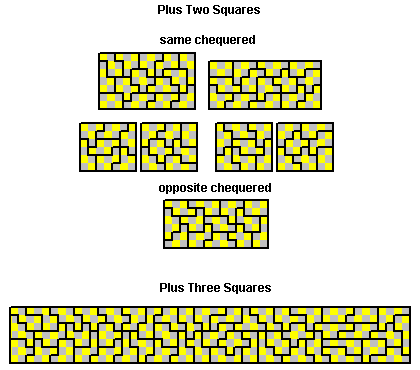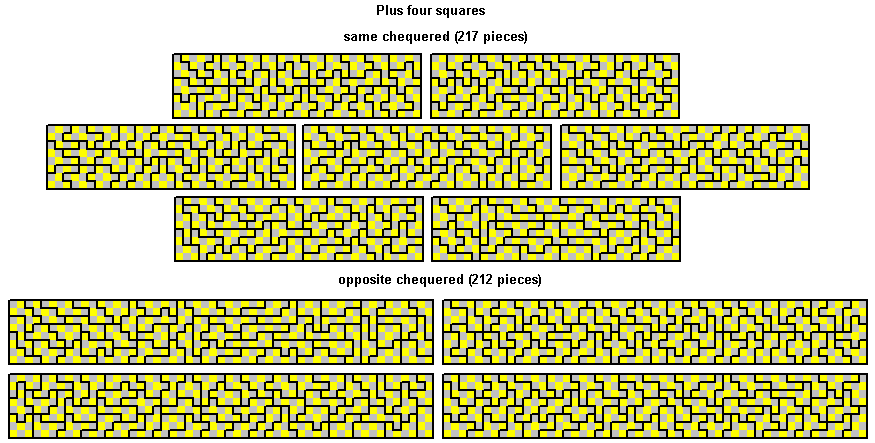Sets Based on the Square Tetromino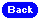Sets Formed by Adding Squares to a Square Tetromino

The eight pieces formed by adding two squares are unbalanced in total on a chessboard colouring and so cannot form a rectangle.The one-sided set of 12 pieces can form rectangles.The twelve piece one-sided set can form rectangles.The 25 pieces in the plus three set can form 5x35 and 7x25 rectangles. A number of other symmetric constructions can be made as well as single and multiple replications of the pieces.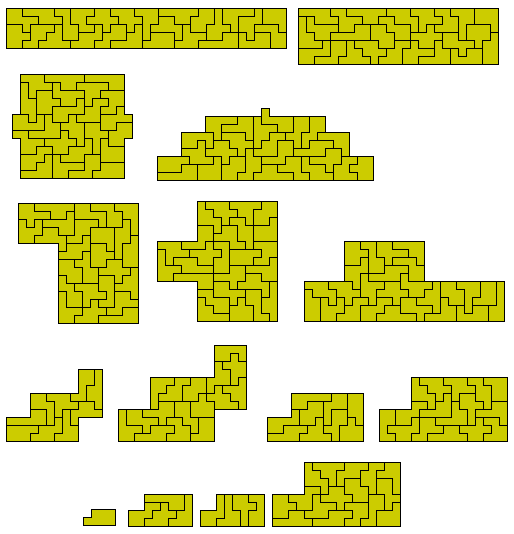The one-sided set consists of 48 pieces and mutiple rectangles can be made.The set formed by adding four squares can for an 8x113 rectangle. It is also possible to make a sets of similar figures with this set based on 131=22+32+52+52+52+52 and 131=12+22+32+32+42+52+72. Other solutions should also be possible based on this, 131=12+22+62+62+62 or other dissections of 131.The one-sided set consists of 212 pieces.The set can also make four 8x53 rectangles.A number of sets of similar figures should also be possible. This one is based on 212=22+32+32+42+52+62+72+82.Another here based on 212=12+52+52+52+62+102.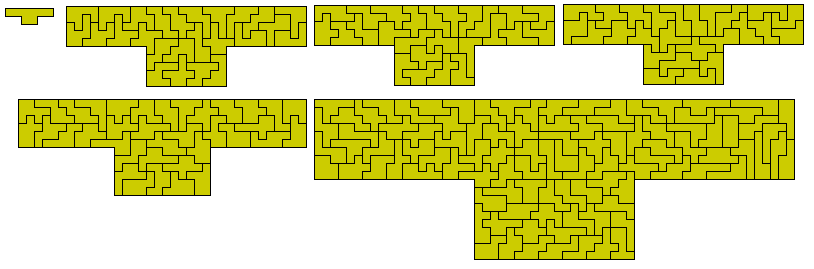and a set of 13 another here based on 212=12+22+2x32+4x42+5x52.A set of 23 based on 212=12+3x22+15x32+4x42 would seem very unlikely.

Sets Formed by Adding Dominoes to a Square Tetromino
The 40 pieces formed by adding two dominoes has a total area of 320 unit squares and can make simultaneous rectangles.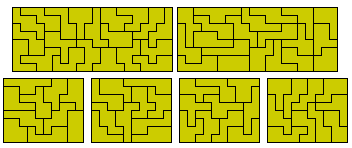Although the total area of this set is that of five side 8 squares such a construction cannot be made owing to the lack of pieces which can form the edges. Five congruent figures are however possible.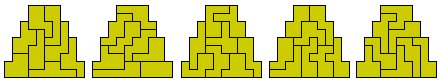Sets of similar figures can also be made with the set.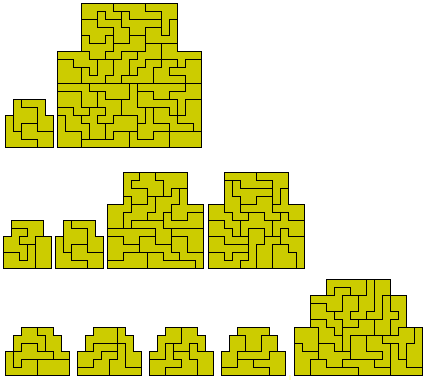Other symmetric constructions are also possible.The one-sided set consists of 71 pieces and one rectangle is possible.Sets Formed by Adding a Square Tetromino to the Trominoesone-sided setSets Formed by Adding a Square Tetromino to the Tetrominoes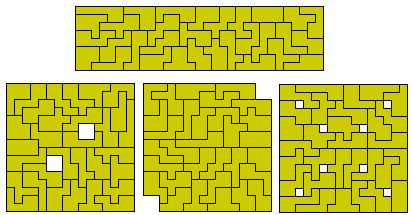one-sided setSets Formed by Adding a Square Tetromino to the Pentominoes.
There are 109 pieces in this set including one piece with a hole. Omitting this piece a 9x108 rectangle can be made.Finally a few solutions for the coresponding chequered sets.